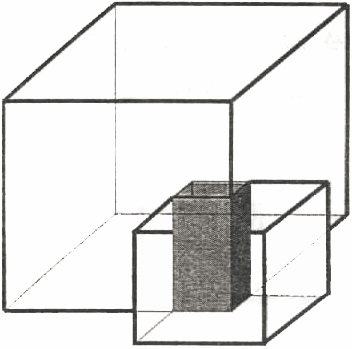## Day 2, Problem 3 - Satellite Coverage

SERCOI (Space-Earth Resource Cover-Observe Institute) is an organization committed to using satellite technology and the Earth's resources for observation and research. They have successfully built a new satellite model, the SERCOI-308. This satellite can provide coverage to a cube-shaped region of a certain volume while being situated at the cube's center.The Cartesian co-ordinates (x, y, z) denote its position at the center of the cube, and r denotes the distance from its position, the center, to any face of the cube (thus, r is half the height of the cube). The edges of the cube are parallel to their respective axes. We can therefore use the 4-tuple (x, y, z, r) to describes the state of any such satellite. Thus, the volume that this satellite can cover is V = (2r)3 = 8r3.

Since the region that any satellite can cover is limited, multiple satellites can work together to cover certain regions. The spaces where they can cover may overlap, as shown in the given diagram (the shaded sections indicate overlapping coverage).

Please write a program that, given the states of many satellites, calculates the total volume being covered.

### Input Format

The first line of input contains one integer N (1 ≤ N ≤ 100), the number of satellites. The following N lines describe the states of the N satellites. Each of these lines will contain four space-separated integers x, y, z, and r (−1000 ≤ x, y, z ≤ 1000, 1 ≤ r ≤ 200), the co-ordinates of the satellite and its half-height.

### Output Format

The output should contain one line with one integer, the total volume covered by all of the satellites.

```3
0 0 0 3
1 -1 0 1
19 3 5 6
```

### Sample Output

`1944`

Point Value: 17 (partial)
Time Limit: 1.00s
Memory Limit: 64M